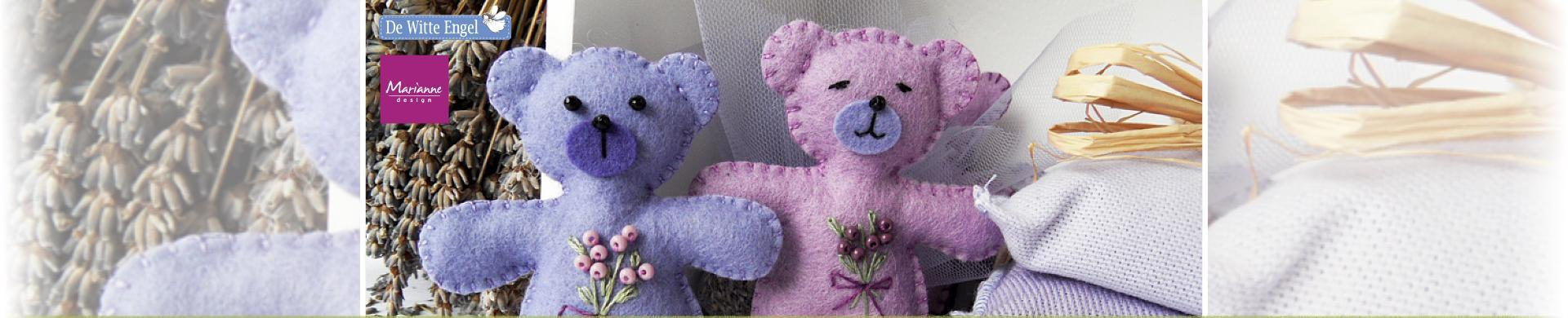# De Witte EngelDe Witte Engel is located in The Netherlands and established in 1990. De Witte Engel TrueFelt is a 1.2mm thick, 100% wool felt fabric, ideal for making dolls and toys, items for your home and so very much more. When cutting felt using cutting dies, the best results will be achieved with TrueFelt.
• Brand
• Colour
1. \$2.38
2. \$2.38
3. \$2.38
4. \$2.38
5. \$2.38
6. \$9.32
7. \$8.28
8. \$2.38
9. \$2.38
10. \$3.72
11. \$4.13
12. \$4.13
13. \$4.13
14. \$4.13
15. \$4.13
16. \$4.13
17. \$2.38
18. \$2.38
19. \$2.38
20. \$2.38
21. \$2.38
22. \$2.38
23. \$2.38
24. \$2.38
25. \$2.38
26. \$2.38
27. \$2.38
28. \$2.38
29. \$2.38
30. \$2.38
31. \$2.38
32. \$2.38
33. \$2.38
34. \$2.38
35. \$2.38
36. \$2.38
37. \$2.38
38. \$2.38
39. \$2.38
40. \$2.38
41. \$2.38
42. \$2.38
43. \$2.38
44. \$2.38
45. \$2.38
46. \$2.38
47. \$2.38
48. \$2.38
49. \$2.38
50. \$2.38
51. \$2.38
52. \$2.38
53. \$2.38
54. \$2.38
55. \$2.38
56. \$2.38
57. \$2.38
58. \$2.38
59. \$2.38
60. \$2.38
61. \$2.38
62. \$2.38
63. \$2.38
64. \$2.38
65. \$2.38
66. \$2.38
67. \$2.38
68. \$2.38
69. \$2.38
70. \$2.38
71. \$2.38
72. \$2.38
73. \$2.38
74. \$2.38
75. \$2.38
76. \$2.38
77. \$2.38
78. \$2.38
79. \$2.38
80. \$2.38
81. \$2.38
82. \$2.38
83. \$2.38
84. \$2.38
85. \$2.38
86. \$2.38
87. \$2.38
88. \$2.38